New update is available. Click here to update.
Last Updated: May 27, 2023
Medium

# Kruskal's AlgorithmAuthor Shreya Deep0 upvotes

## Introduction

A Graph is a data structure consisting of edges connecting vertices. When all the vertices of a graph are connected such that there is no cycle and the number of edges is (v-1)  ( where v is the number of vertices of the graph), the weighted tree formed is called a spanning tree

A single graph can have more than one spanning tree, and the spanning tree with the minimum sum of all edge weights is called the minimum spanning tree

There are various algorithms to find the minimum spanning tree of a graph. One such algorithm is Prims Algorithm. In this article, we’ll learn about another algorithm to find the minimum spanning tree. This algorithm is known as Kruskal’s minimum spanning tree.

So let’s get started!

## Problem Statement

The problem states that “Given a connected and undirected graph, find its minimum spanning tree using Kruskal’s minimum spanning tree algorithm.”

Note: A graph having bidirectional edges is known as an undirected graph. Such graphs can be traversed in either direction.

Let’s understand the problem statement with the help of an example.

Given a graph having Number of vertices(V) = 5, and Number of edges(E) = 6. Cost of an edge and the vertices having the edge are(cost, vertex1, vertex2): ( 2,0,1) , (1,4,3), (3,1,2), (2,4,2), (3,2,3), (1,1,4).

The graph contains five vertices and six edges. So the minimum spanning tree will have four edges. The diagram below shows the graph and its minimum spanning tree.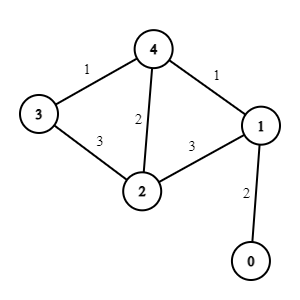Above is the graph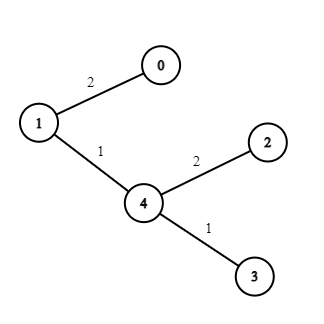Above is the corresponding minimum spanning tree

Edges of the minimum spanning trees are: (1,4), (4,3), (0,1), (4,2). And, the total weight of the tree will be 6.

Now, let's move on to the solution approach of this problem.

## Solution Approach

Kruskal’s minimum spanning tree algorithm is a greedy approach(see Greedy Algorithms)because we pick the smallest edge. We're making that choice because we want our spanning tree to have a minimum total sum of edges.

A graph may or may not contain a cycle. But in MSP, we need to ensure that there is no cycle. So, in this algorithm, we're using a disjoint data structure to find if two nodes are in the same subset or not. This will help detect a cycle

Want to know what a disjoint set data structure is?

• A disjoint set data structure is used to track the division of elements into different disjoint subsets.
• The union-find algorithm performs two operations on this data structure, namely, find and union. For more details, you can refer to this blog.

The steps of Kruskal’s algorithm are as follows :

``````1. Sort all the edges in ascending order based on their weights.

2. Draw the edge with the least weight. Check if it generates a cycle with the spanning-tree formed till now using a union-find algorithm. If the cycle is not formed, include this edge. Else, discard it and move to the next.

3. Repeat step 2 until there are (v-1) edges in the spanning tree. ``````

Let’s understand this algorithm using the above example :The graph contains five vertices and six edges. So, the minimum spanning tree will have (5-1) = four edges. We'll be performing one step at a time and understand how that'll work.

Step 1:  After sorting the edges in increasing order of their weights, we get :

Step 2: Now, we start picking edges one by one until we get four edges of our spanning tree. Currently, our spanning tree has no edges.

• Pick edge 1 - 4: It doesn't form any cycle, so add it.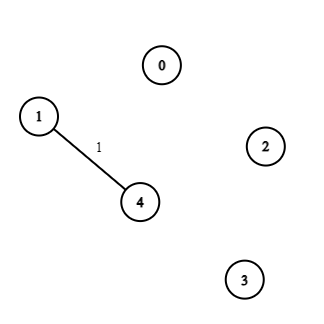• Pick edge 4-3: It doesn't form any cycle, so add it.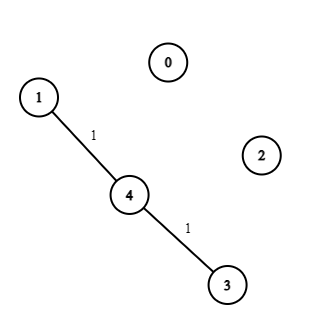• Pick edge 0-1: It doesn't form any cycle, so add it.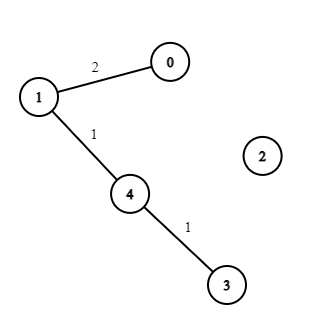• Pick edge 4-2: It doesn't form any cycle, so add it.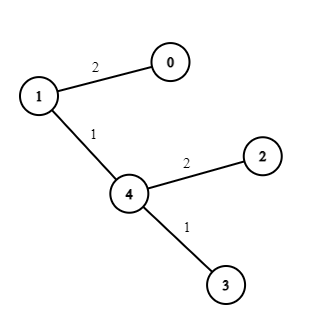At this point, we've already taken four edges in our spanning tree, and therefore, we stop, and our spanning tree is complete and has the minimum weight.

We have taken the edges 1 - 4, 4 - 3, 0 - 1, and 4 - 2. Thus, the total weight of the minimum spanning tree is 1+1+2+2 = 6

You’ve now understood the working and the reasoning behind using Kruskal’s minimum spanning tree algorithm to find the minimum spanning tree.

Let’s work on the implementation now!

Before directly jumping to the solution, we suggest you try and solve this problem on CodeStudio.

### Implementation

Let’s see the implementation of the above approach.

``````#include<bits/stdc++.h>

using namespace std;

const int MAX = 1e6-1;

int parent[MAX];

int find(int a){  //function to find the parent of the subset this a belongs to

while(parent[a]!=a){

parent[a] = parent[parent[a]];

a = parent[a];

}

return a;

}

void union_(int a,int b){  //function to merge two subsets

int d = find(a);

int e = find(b);

parent[d] = parent[e];
}

int main(){

int vertices;

cin>>vertices;

int edges;

cin>>edges;

vector<pair<int,pair<int,int>>>adj;      // vector to store the edges in the form - > {weight, {source, destination}}

for(int i=0;i<edges;i++){

int weight;

int src,destination;

cin>>weight>>src>>destination;

adj.push_back({weight,{src,destination}});   // pushing back the edges one by one

}

for(int i = 0;i<MAX;i++){

parent[i] = i;        // initialising the parent of each node as itself

}

vector<pair<int,int>>tree_edges;      // vector for storing the edges of the minimum spanning tree

int totalweight = 0;     //initialising the total weight to 0

int a = x.second.first;

int b = x.second.second;

int cost = x.first;

if(find(a)!=find(b)){        // if the two vertices are in different subsets, merge them into one

totalweight+=cost;

union_(a,b);

tree_edges.push_back({a,b});

}

}

cout<<"Edges are : "<<endl;

for(auto x:tree_edges){  // printing the edges of the minimum spanning tree

cout<<x.first<<" "<<x.second<<endl;

}

cout<<"Total weight of MST = ";

cout<<totalweight<<endl;        //printing the total weight of the minimum spanning tree

return 0;

}``````

Input

``````//entering the number of vertices

5

//entering the number of edges

6

//entering edges one by one in the format: cost, v1, v2

2 0 1

1 4 3

3 1 2

2 4 2

3 2 3

1 1 4``````

Output

``````//Output

Edges are :

1 4

4 3

0 1

4 2

Total weight of MST = 6``````

### Complexity Analysis

Time Complexity

The time complexity of Kruskal's minimum spanning tree algorithm is O(E*logV), where E is the number of edges in the graph and V is the number of vertices.

Reason: Since we're sorting the edges, which takes O(E*logE) time. Then we check for each edge whether to add it or not by using the union-find algorithm, which takes at most O(logV) time for every edge E, Hence total O(ElogV).

Thus the overall complexity will be O(ElogE + ElogV), which is approximately O(ElogV).

Space Complexity

The space complexity of Kruskal's minimum spanning tree algorithm is O(|E| + |V|), where E is the number of edges in the graph and V is the number of vertices.

Reason: Since the disjoint set structure takes O(|V|) space to store the parents of vertices and O(|E|), we’re additionally storing the edges of the graph.

If you've made it this far, congratulations, Champ! You've understood the Kruskal’s minimum spanning tree algorithm”. If you haven't already submitted it to CodeStudio. Without further ado, have it accepted as early as possible.

You must watch this video for the conceptual understanding and proper code implementation of the “Kruskal’s Algorithm”.

### What is Kruskal’s minimum spanning tree?

The tree formed using Kruskal's minimum spanning tree algorithm on a graph is called the minimum spanning tree.

### What is a spanning tree?

A spanning tree of a Graph G is its subset, having all the vertices covered with the minimum possible number of edges. Thus, a spanning tree has v-1 edges.

### What is a minimum spanning tree?

A spanning tree with a minimum sum of all edges among all the spanning trees of a graph is called the minimum spanning tree.

### What is Kruskal’s algorithm used for?

Kruskal’s algorithm is used for finding the minimum spanning tree of a graph.

### What are minimum spanning trees used for?

For network architectures, minimum spanning trees are utilized (i.e. telephone or cable networks). They're also utilized to develop approximate solutions to complex mathematical issues like the Traveling Salesman Problem.

## Conclusion

In this article, we've discussed Kruskal’s minimum spanning tree algorithm here, along with its approach and implementation in C++.

Other similar algorithms are Prim’s algorithm using a minimum spanning tree and The traveling salesman problem. Don't forget to try it out, as it'll help you empower your understanding.Go on top